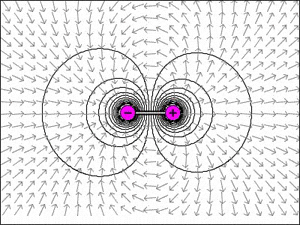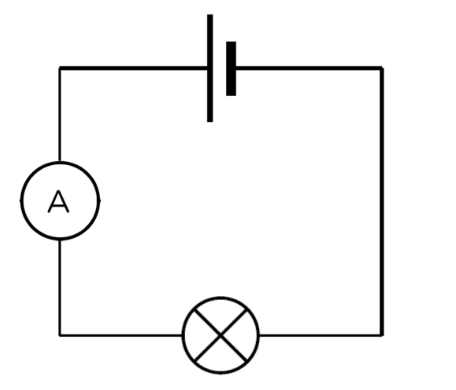Search

# Electric fields

Updated: Oct 23, 2019

Hi! In order to understand this blog post, please read the previous one, Electric fields. In this post I will talk about Coulomb's law, electric current and energy and charge.Coulomb’s law allows us to calculate the electric force between the electric force between two point charges. A force of equal magnitude acts on both charges, but in opposite directions.

The magnitude of the force, F, between two isolated point charges, q1 and q2, is proportional to the product of the charges and inversely proportional to the square of the distance, r, between them.

The equation is F = k q1 q2 / r^2 where k is Coulomb's constant and the value is 8.99 * 109 N m^2 C^-2. The electric charge of a particular medium is represented by its permittivity, ε.

An electric current is a flow of charged particles. In a electrical circuit the moving charge is most often electrons. Current is given the symbol I.

Current = charge / time, I = Δ q / Δt . The unit of current is ampere(amp), A. 1 A = 1 C s^-1.

A material where charge can flow through is called a conductor. A material where charge can’t flow through is called an insulator. The difference between those two are the amount of free electrons. In a good conductor such as metals free electrons can move freely around. Electric current is measured by amme

ter. In Figure 1, the ammeter is connected to a lamp and a cell.Figure 1

The drift speed, v, of electrons or other charge carriers in a current is their net speed along the conductor. Since metals contain free electrons that move around randomly. An electric current is created when those electrons are made to drift along a wire the same direction. The drift speed equation, I = nAvq can be derived from the current equation.

Energy can be transferred to or from charges. The energy that would be transferred if one unit of charge, 1C was moved between two points is called the potential difference, p.d, V, between those two points. p.d. = energy transferred (work done) / charge. V = W/q. The unit for p.d. (voltage) is Volt. This concept of potential difference can also be applied to any electric field.

An unit for energy in atomic physics is the electronvolt. It is used because the charge for electrons is small. It is defined as the unit of energy which is equal to what is transferred when a charge of 1.6 * 10-19 C moves through a p.d. of 1 V.

Figure 1; http://www.mstworkbooks.co.za/natural-sciences/gr8/gr8-ec-03.html

Sources: Physics for the IB diploma, third edition, John Allum and Christopher Talbot